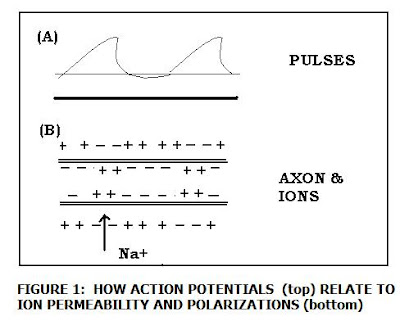## Tuesday, July 20, 2010

### Numerical Testing of the MLP Network MappingBefore going on to the subject of the numerical tests for a multilayer perceptron network applied to the brain's amygdala, let's briefly summarize one of the key points from the previous blog. That is, how "waves of polarization" travel along the axons to generate signals and impulses which will affect target neurons at the putative outputs.

In Figure 1, I show simultaneously a given wave of polarization in the axon and the distribution of charges (+,-) that would incept the action potential. Note that each of the pulse peaks (A) correlate to a biased concentration of Na+ ions (over K+) in the axon and the emergence of many more (-) charges associated with the negatively charged protein molecules inside the cells. The converse is true where the action potential minima occur. What we discussed then was whether any way existed (using neural networks in the region of interest) by which the charge distribution could suddenly be shifted in order to shut down the traveling impulse and yield a null output.

We considered two neural map networks: 1) the Kohonen SOM, and 2) the Multilayer Perceptron network. We decided that (1) couldn't fill the bill for a variety of reasons, but mainly it was oversimplified and there was no scope to implant devices for bias, such as we might find in a (Pauli spin matrix function) quantum dot logic gate that flips an output (0,1) say, to (0, -1). We then began to examine the MLP network to see if it offered anything better.

To recap briefly: There are n neurons in the input layer, p neurons in the hidden layer, and m neurons in the output layer. The weights between the input layer and the hidden layer are labelled (V_ij), and the weights between the hidden and the output layers are to be labelled (W_jk) , where 0 less than or equal to i less than or equal to n, and the input "training" vector x_ij = {x1, x2, x3, x_n}. In the numerical experiment we will be trying to recover three key quantities: Z(i,j), the total input stimuli to a neuron in the hidden layer (nth neuron matched to x_n training vector), y(i,j) the total input stimuli to a neuron in the output layer, and y(k=j) the output of a neuron in the output layer.

To simplify the computations using Mathcad (14), and also to avoid violating Mathcad's numerical computation rules and limits, I allowed the indices to range over the same (limited) number of neurons so: i= j = k, which allowed a more simplified summation. The equations used are shown in Fig. 2, as well as results from computing Z(i,j). I enumerated over the first 50 neurons, so i = j = k = 50. The table shows the partial results given in volts. Fig. 2 also shows the choice of the other parameters to compute Z(i,j) which were determined the most plausible to be required given the limitations of the map.

It is noted that while the input stimuli for Z(i,j) were within bounds here, the resulting total input stimuli to neurons in the output layer was zero. (Or in other words, so small the value or magnitude of the voltage became insignificant). Now, since the ouput of a neuron in the output layer is a function of the input stimuli to neurons in the output layer: e.g. y(k=j) = f(y(i,j)) then we also have y(k=j) = 0. Superficially this (zero) output looks like just what we're seeking, but not so fast! We did not want it at the cost of simply have y(i,j) = 0 (e.g. 0 input signal to neurons in the output layer.)

Thus, it appears that our project to alter the brain dynamics of fundamentalists will have to consider other, perhaps more detailed, neural networks. When we do arrive at one that works, we can then recommend the best quantum dot gate for implantation in the resident brain - to offset their "Hell" beliefs, intolerance and other detritus.